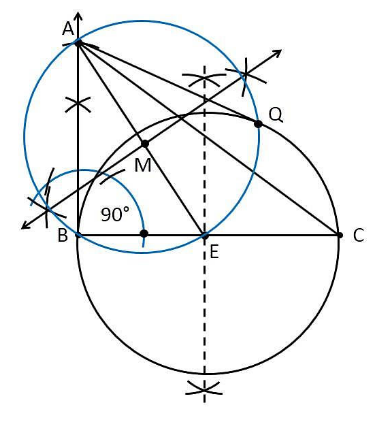Guru

# Let ABC be a right triangle in which AB = 6 cm, BC = 8 cm and ∠ B = 90°. BD is the perpendicular from B on AC. The circle through B, C, D is drawn. Construct the tangents from A to this circle. Q.6

• 0

I am not able to solve this problem sir please help me for solving this problem give me the best and the easy way for the question of class 10th ncert math of exercise 11.2 of constructions of ncert math Let ABC be a right triangle in which AB = 6 cm, BC = 8 cm and ∠ B = 90°. BD is the perpendicular from B on AC. The circle through B, C, D is drawn. Construct the tangents from A to this circle.

Share

1. Construction Procedure:

The tangent for the given circle can be constructed as follows

1. Draw the line segment with base BC = 8cm

2. Measure the angle 90° at the point B, such that ∠ B = 90°.

3. Take B as centre and draw an arc with a measure of 6cm.

4. Let the point be A where the arc intersects the ray.

5. Join the line AC.

6. Therefore, ABC be the required triangle.

7. Now, draw the perpendicular bisector to the line BC and the midpoint is marked as E.

8. Take E as centre and BE or EC measure as radius draw a circle.

9. Join A to the midpoint E of the circle

10. Now, again draw the perpendicular bisector to the line AE and the midpoint is taken as M

11. Take M as Centre and AM or ME measure as radius, draw a circle.

12. This circle intersects the previous circle at the points B and Q

13. Join the points A and Q

14. Therefore, AB and AQ are the required tangentsJustification:

The construction can be justified by proving that AG and AB are the tangents to the circle.

From the construction, join EQ.

∠AQE is an angle in the semi-circle. We know that an angle in a semi-circle is a right angle.

∴ ∠AQE = 90°

⇒ EQ⊥ AQ

Since EQ is the radius of the circle, AQ has to be a tangent of the circle. Similarly, ∠B = 90°

⇒ AB ⊥ BE

Since BE is the radius of the circle, AB has to be a tangent of the circle.

Hence, justified.

• 0